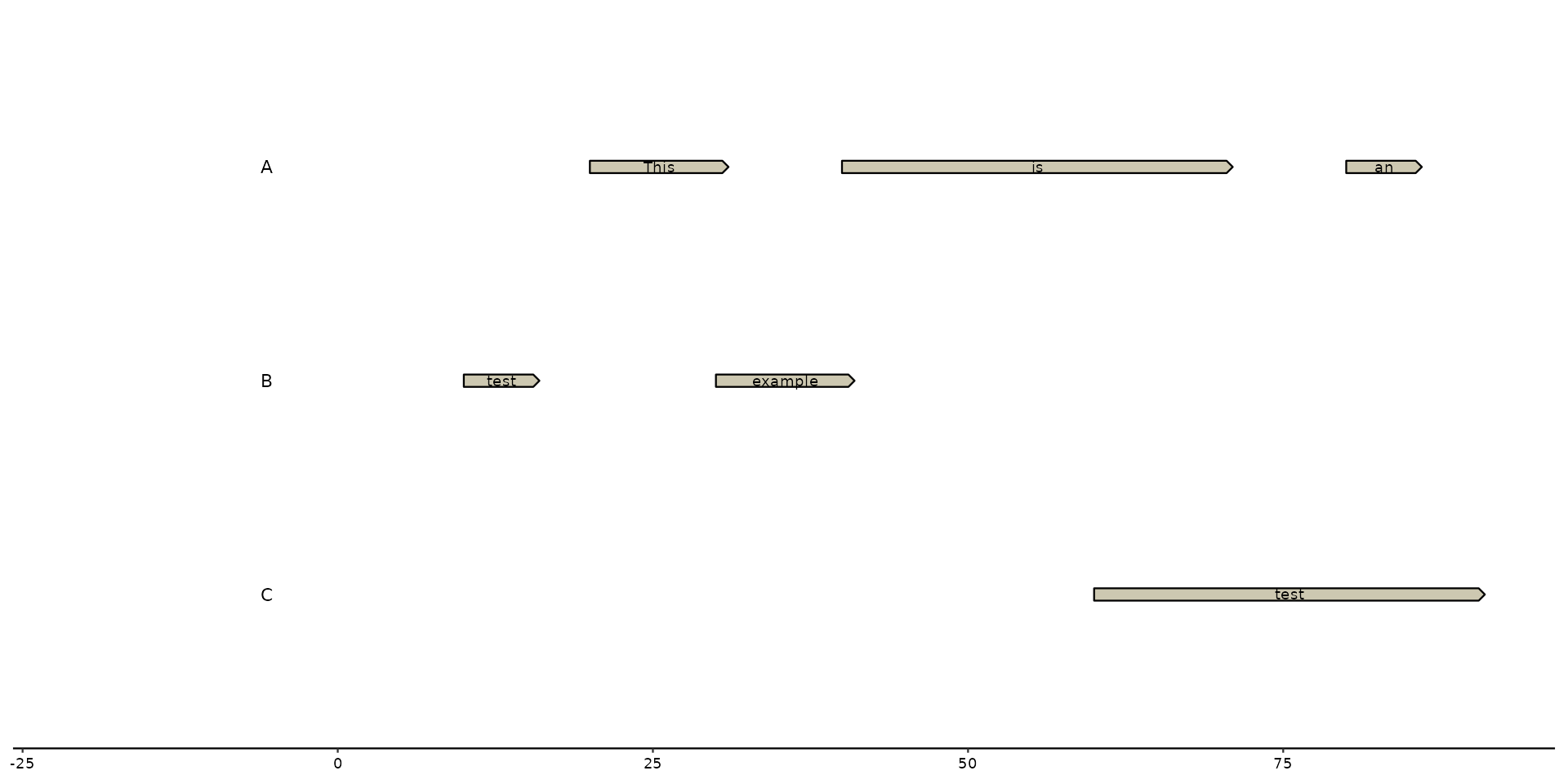The functions below are useful for labeling features/genes in plots. Users have to call on aes(label = ...) or (label = ...) to define label's text Based on the function, the label will be placed at a specific location:

• geom_..._text() will plot text in the middle of the feature.

• geom_..._tag() will plot text on top of the feature, with a 45 degree angle.

• geom_..._note() will plot text under the feature at the left side.

The ... can be either replaced with feat or gene depending on which track the user wants to label.

With arguments such as hjust, vjust, angle, and nudge_y, the user can also manually change the position of the text.

geom_feat_text(
mapping = NULL,
data = feats(),
stat = "identity",
position = "identity",
...,
parse = FALSE,
check_overlap = FALSE,
na.rm = FALSE,
show.legend = NA,
inherit.aes = TRUE
)

geom_feat_tag(
mapping = NULL,
data = feats(),
stat = "identity",
position = "identity",
hjust = 0,
vjust = 0,
angle = 45,
nudge_y = 0.03,
xjust = 0.5,
strandwise = TRUE,
...,
parse = FALSE,
check_overlap = FALSE,
na.rm = FALSE,
show.legend = NA,
inherit.aes = TRUE
)

geom_feat_note(
mapping = NULL,
data = feats(),
stat = "identity",
position = "identity",
hjust = 0,
vjust = 1,
nudge_y = -0.03,
xjust = 0,
strandwise = FALSE,
...,
parse = FALSE,
check_overlap = FALSE,
na.rm = FALSE,
show.legend = NA,
inherit.aes = TRUE
)

geom_gene_text(
mapping = NULL,
data = genes(),
stat = "identity",
position = "identity",
...,
parse = FALSE,
check_overlap = FALSE,
na.rm = FALSE,
show.legend = NA,
inherit.aes = TRUE
)

geom_gene_tag(
mapping = NULL,
data = genes(),
stat = "identity",
position = "identity",
hjust = 0,
vjust = 0,
angle = 45,
nudge_y = 0.03,
xjust = 0.5,
strandwise = TRUE,
...,
parse = FALSE,
check_overlap = FALSE,
na.rm = FALSE,
show.legend = NA,
inherit.aes = TRUE
)

geom_gene_note(
mapping = NULL,
data = genes(),
stat = "identity",
position = "identity",
hjust = 0,
vjust = 1,
nudge_y = -0.03,
xjust = 0,
strandwise = FALSE,
...,
parse = FALSE,
check_overlap = FALSE,
na.rm = FALSE,
show.legend = NA,
inherit.aes = TRUE
)

## Arguments

mapping

Set of aesthetic mappings created by aes(). If specified and inherit.aes = TRUE (the default), it is combined with the default mapping at the top level of the plot. You must supply mapping if there is no plot mapping.

data

The data to be displayed in this layer. There are three options:

If NULL, the default, the data is inherited from the plot data as specified in the call to ggplot().

A data.frame, or other object, will override the plot data. All objects will be fortified to produce a data frame. See fortify() for which variables will be created.

A function will be called with a single argument, the plot data. The return value must be a data.frame, and will be used as the layer data. A function can be created from a formula (e.g. ~ head(.x, 10)).

stat

The statistical transformation to use on the data for this layer, either as a ggproto Geom subclass or as a string naming the stat stripped of the stat_ prefix (e.g. "count" rather than "stat_count")

position

Position adjustment, either as a string, or the result of a call to a position adjustment function. Cannot be jointly specified with nudge_x or nudge_y.

...

Other arguments passed on to layer(). These are often aesthetics, used to set an aesthetic to a fixed value, like colour = "red" or size = 3. They may also be parameters to the paired geom/stat.

parse

If TRUE, the labels will be parsed into expressions and displayed as described in ?plotmath.

check_overlap

If TRUE, text that overlaps previous text in the same layer will not be plotted. check_overlap happens at draw time and in the order of the data. Therefore data should be arranged by the label column before calling geom_text(). Note that this argument is not supported by geom_label().

na.rm

If FALSE, the default, missing values are removed with a warning. If TRUE, missing values are silently removed.

show.legend

logical. Should this layer be included in the legends? NA, the default, includes if any aesthetics are mapped. FALSE never includes, and TRUE always includes. It can also be a named logical vector to finely select the aesthetics to display.

inherit.aes

If FALSE, overrides the default aesthetics, rather than combining with them. This is most useful for helper functions that define both data and aesthetics and shouldn't inherit behaviour from the default plot specification, e.g. borders().

hjust

Moves the text horizontally

vjust

Moves the text vertically

angle

Defines the angle in which the text will be placed. *Note

nudge_y

Moves the text vertically an entire contig/sequence. (e.g. nudge_y = 1 places the text to the contig above)

## Details

These labeling functions use ggplot2::geom_text() under the hood. Any changes to the aesthetics of the text can be performed in a ggplot2 manner.

## Examples

# example data
genes <- tibble::tibble(
seq_id = c("A", "A", "A", "B", "B", "C"),
start = c(20, 40, 80, 30, 10, 60),
end = c(30, 70, 85, 40, 15, 90),
feat_id = c("A1", "A2", "A3", "B1", "B2", "C1"),
type = c("CDS", "CDS", "CDS", "CDS", "CDS", "CDS"),
name = c("geneA", "geneB", "geneC", "geneA", "geneC", "geneB"))

seqs <- tibble::tibble(
seq_id = c("A", "B", "C"),
start = c(0,0,0),
end = c(100, 100, 100),
length = c(100, 100, 100))

# basic plot creation
plot <- gggenomes(seqs=seqs, genes=genes) +
geom_bin_label() +
geom_gene()

# geom_..._text
plot + geom_gene_text(aes(label=name))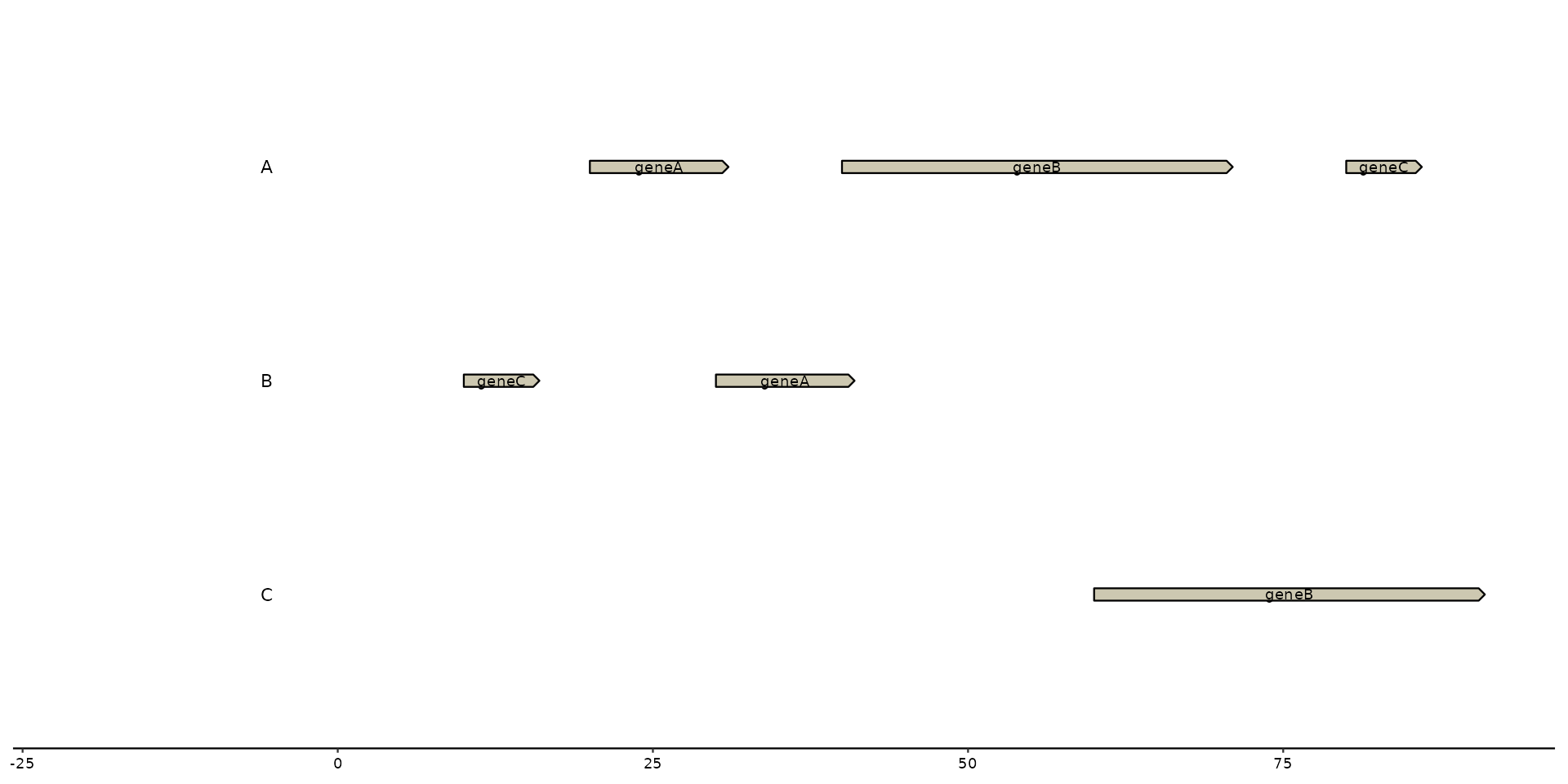# geom_..._tag
plot + geom_gene_tag(aes(label=name))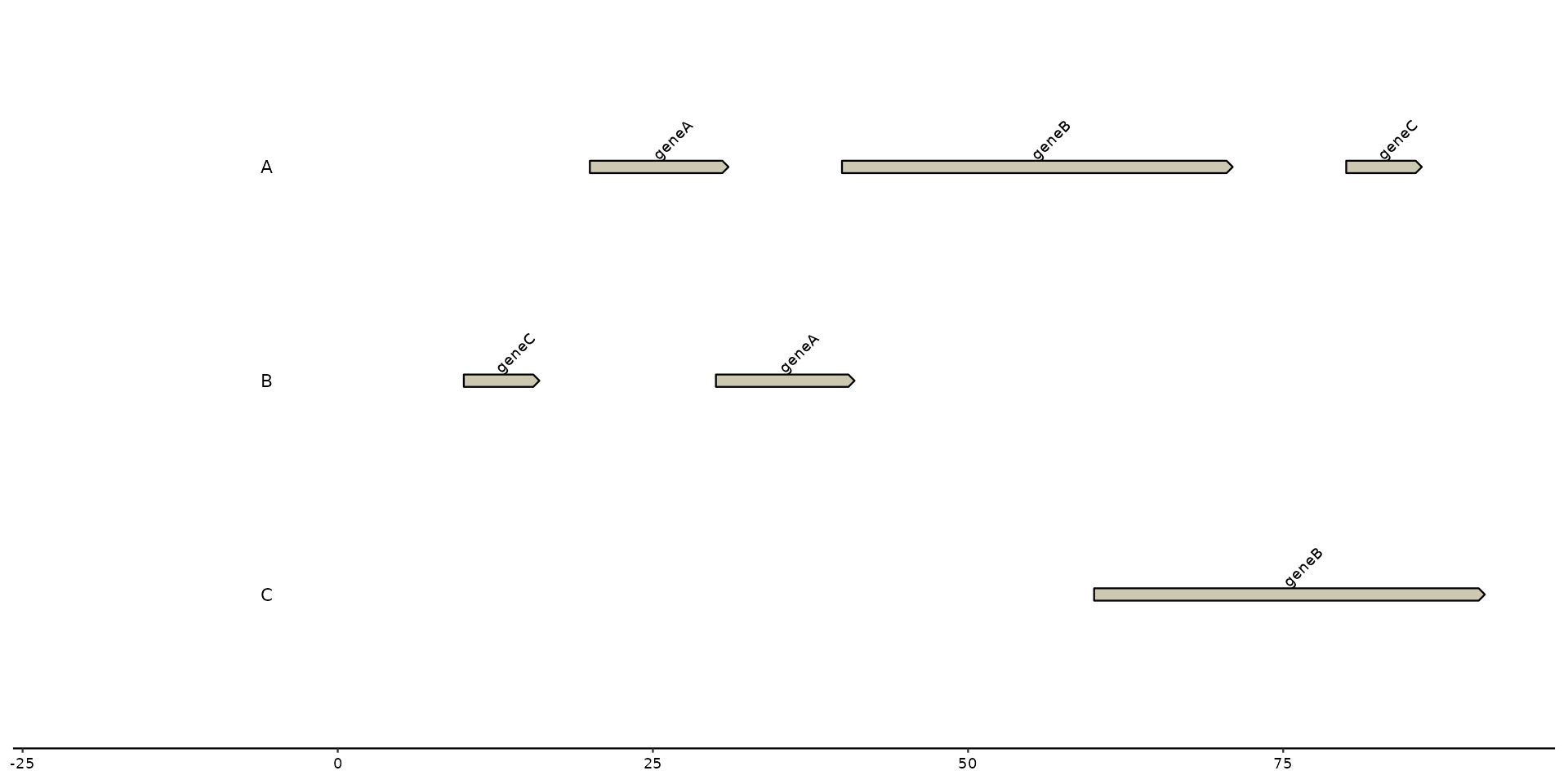# geom_..._note
plot + geom_gene_note(aes(label=name))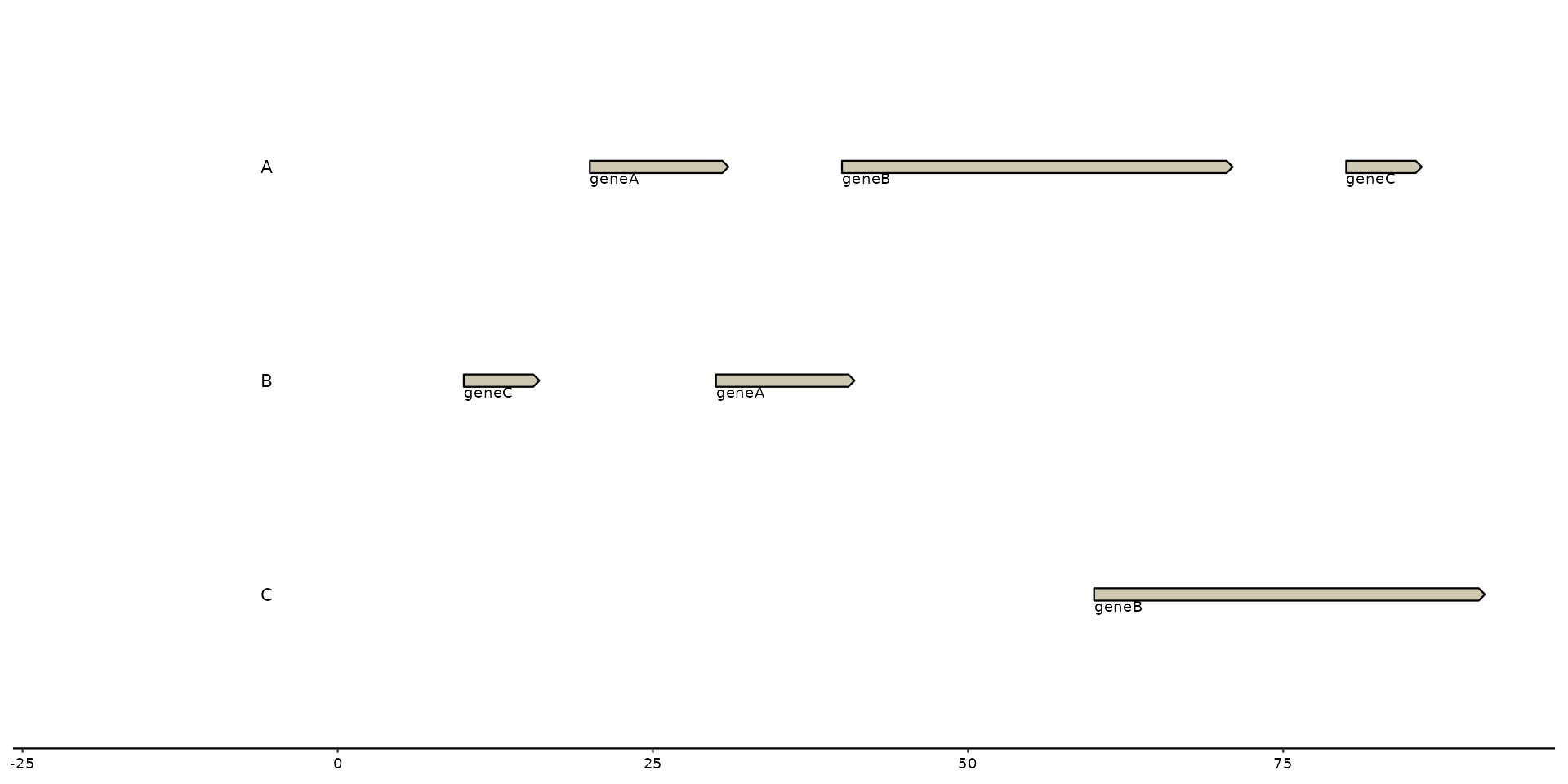# with horizontal adjustment (hjust), vertical adjustment (vjust)
plot + geom_gene_text(aes(label=name), vjust = -2, hjust = 1)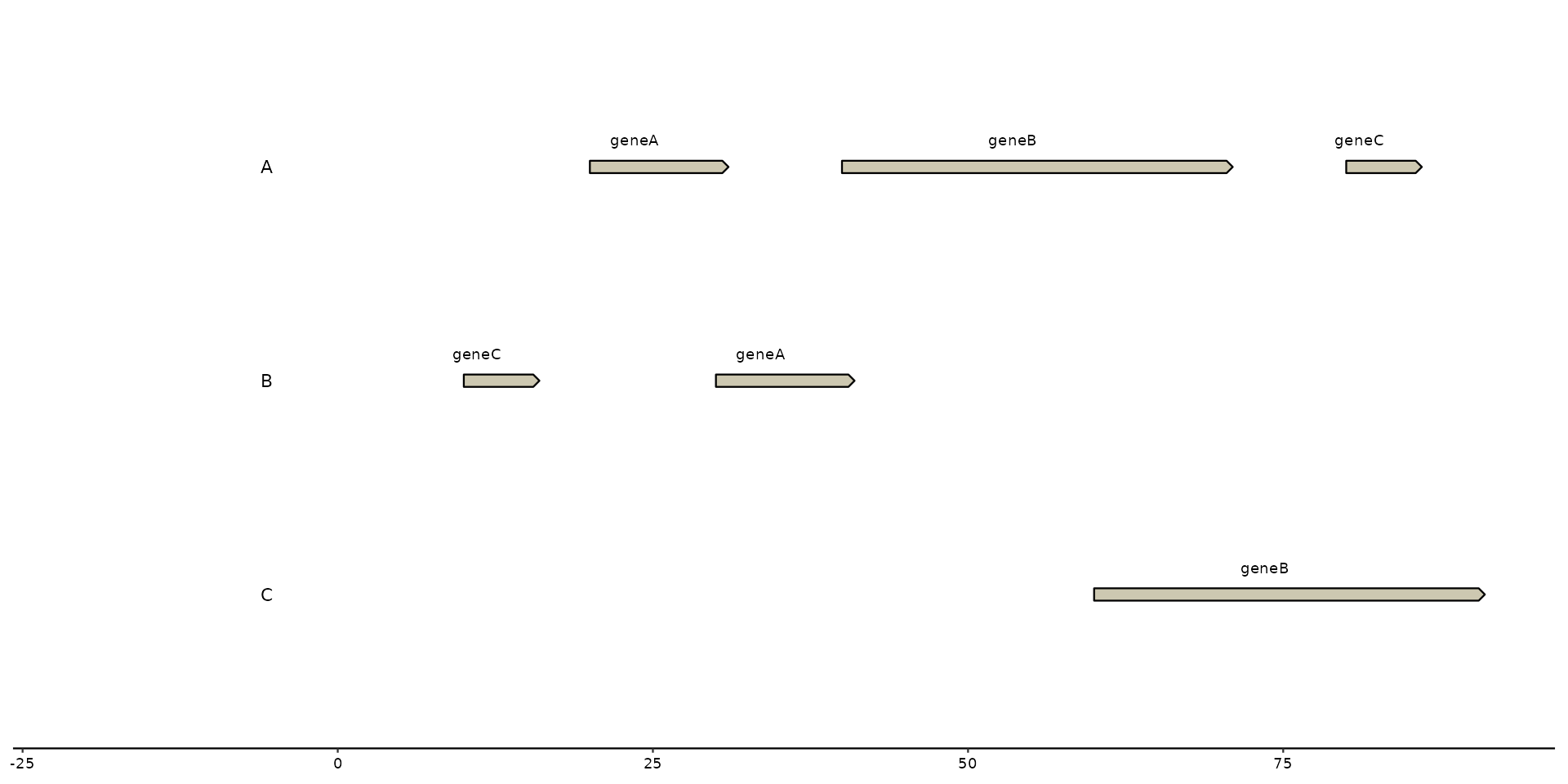# using nudge_y and and angle adjustment
plot + geom_gene_text(aes(label=name), nudge_y= 1, angle = 10)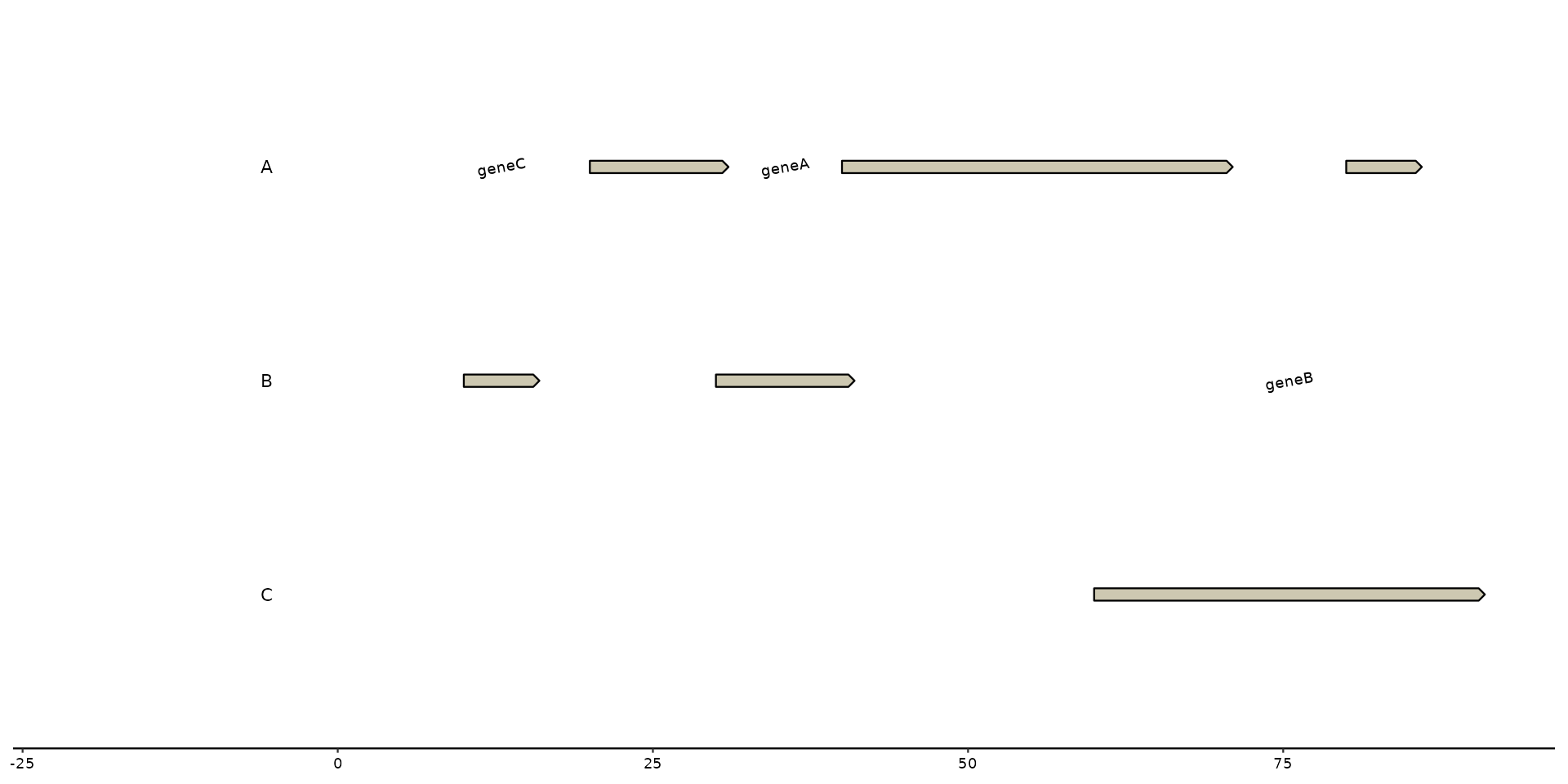# labeling with manual input
plot + geom_gene_text(label = c("This", "is", "an", "example", "test", "test"))